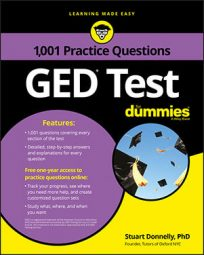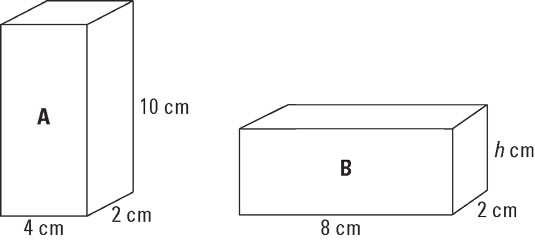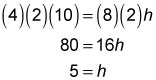##### GED TestThe GED Math test will throw some geometry questions at you that involve three-dimensional shapes like prisms. Usually, they will be in the form of a cube or box, as in the following practice questions.

## Practice Questions

1. A cube has a surface area of 216 square inches. What is its volume in cubic inches?

A. 6 B. 36 C. 108 D. 216

2. If these two boxes have the same volume, what is the height of the second box?A. 4 B. 5 C. 8 D. 10

## Answers and Explanations

1. The correct answer is D.

You can determine the surface area (SA) of a cube with the following equation, in which s is the length of a side:

SA = 6s2

You know the surface area equals 216 cubic inches, so you can substitute this into the equation for SA and solve for s:

216 = 6s2

Dividing both sides by 6 gives you

36 = s2

Hence, s = 6.

A cube is a special type of rectangular prism in which the length (l), the width (w), and the height (h) are all equal. The volume of a rectangular prism is given by

V = lwh

Substituting l = 6, w = 6, and h = 6 into the equation shows the volume equals 216 cubic inches. Hence, Choice (D) is the correct answer.

2. The correct answer is B.

The volume of a box (rectangular prism) is V = lwh, where l is the length, w is the width, and h is the height. Set the volumes of the two boxes equal to each other and solve for h:Therefore, Choice (B) is the correct answer.